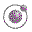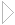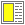Name binding, Recursion, Iteration, and Continuations - slide 42 : 42

Practical example: Searching a binary tree
Searching a binary tree involves a recursively defined tree traversal

If we find the node we are looking for it is convenient to throw the out of the tree traversal

```(define (find-in-tree tree pred)
(call-with-current-continuation
(lambda (found)
(letrec
((find-in-tree1
(lambda (tree pred)
(if (pred tree)
(found tree)
(let ((subtrees (subtree-list tree)))
(for-each
(lambda (subtree) (find-in-tree1 subtree pred))
subtrees)))
#f)))
(find-in-tree1 tree pred)))  ))```